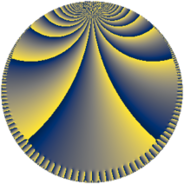# Properties

 Label 252.9.qLevel $252$ Weight $9$ Character orbit 252.q Rep. character $\chi_{252}(143,\cdot)$ Character field $\Q(\zeta_{6})$ Dimension $256$ Sturm bound $432$

# Related objects

## Defining parameters

 Level: $$N$$ $$=$$ $$252 = 2^{2} \cdot 3^{2} \cdot 7$$ Weight: $$k$$ $$=$$ $$9$$ Character orbit: $$[\chi]$$ $$=$$ 252.q (of order $$6$$ and degree $$2$$) Character conductor: $$\operatorname{cond}(\chi)$$ $$=$$ $$84$$ Character field: $$\Q(\zeta_{6})$$ Sturm bound: $$432$$

## Dimensions

The following table gives the dimensions of various subspaces of $$M_{9}(252, [\chi])$$.

Total New Old
Modular forms 784 256 528
Cusp forms 752 256 496
Eisenstein series 32 0 32

## Trace form

 $$256 q + O(q^{10})$$ $$256 q - 55676 q^{16} - 447872 q^{22} - 9362624 q^{25} + 3693340 q^{28} - 4288960 q^{37} - 14414340 q^{40} + 6048816 q^{46} + 18969088 q^{49} + 34461108 q^{52} + 13029596 q^{58} + 24843480 q^{64} + 84129300 q^{70} + 282911040 q^{73} + 101638356 q^{82} + 139238144 q^{85} + 27558404 q^{88} + 237745260 q^{94} + O(q^{100})$$

## Decomposition of $$S_{9}^{\mathrm{new}}(252, [\chi])$$ into newform subspaces

The newforms in this space have not yet been added to the LMFDB.

## Decomposition of $$S_{9}^{\mathrm{old}}(252, [\chi])$$ into lower level spaces

$$S_{9}^{\mathrm{old}}(252, [\chi]) \cong$$ $$S_{9}^{\mathrm{new}}(84, [\chi])$$$$^{\oplus 2}$$